## Maths Tricks That Will Blow Your Mind

Do you also find maths difficult? or should I just simply say do you also find doing calculations difficult? What if I say, I can tell you an easy way to do some maths calculations very easily? Are you looking for ways to do your mathematics calculation in your head? Then, my friend, you are in the right place. Today we are here with “Maths Tricks That Will Blow Your Mind”. These tips are very general and will make mathematics more fun for you. Check out “Maths Tricks That Will Blow Your Mind”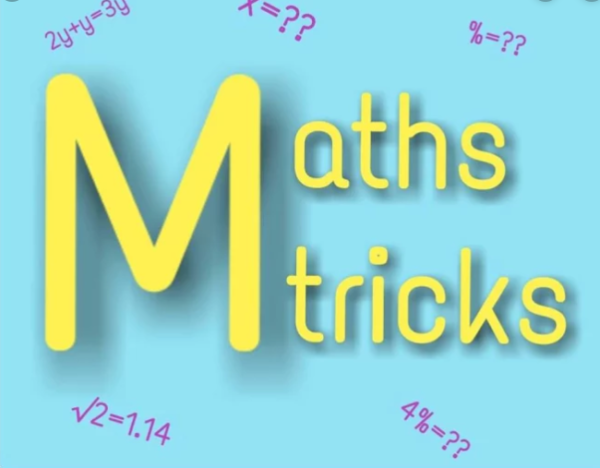## #1. Multiplying By 6

If an even number is multiplied by 6, the answer you’ll get will end with digit you multiplied it with. Also, the digit in tens place will always be half of the digit in the one’s place.

Example- 6 x 4 = 24

Here 4 is an even number, and when we multiplied it by 6, you can see it ended with the same digit i.e. 4. Also, the digit is the tense place i.e 2 is tha half of the digit in one’s place i.e 4.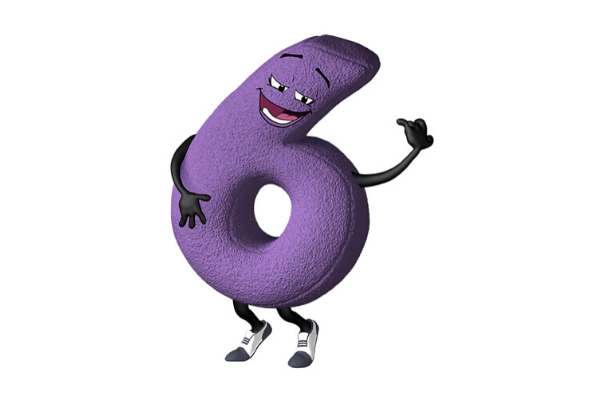## #2. The Answer is 2

1- Take a number, any number you like

2- Multiply the number by 3

4- Divide the answer you get by 3

5- And at last subtract the number you thought in step 1 from the answer you got in step 4

6- You’ll always get the answer as 2

Try it!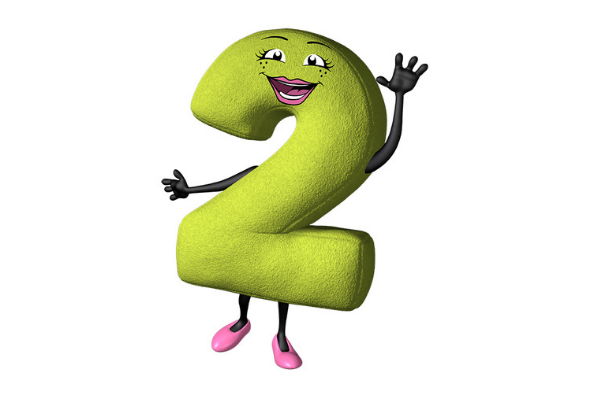## #3. Three-Digit Number

1- Think a three-digit number but, all the three-digit of the number should be the same. For example 222, 555, 888, 666

2- Now, Add up the three digits.

3- And divide the three-digit number you selected by the number you got in step 2.

You’ll see no matter whatever the three-digit number you select the answer will always be 37.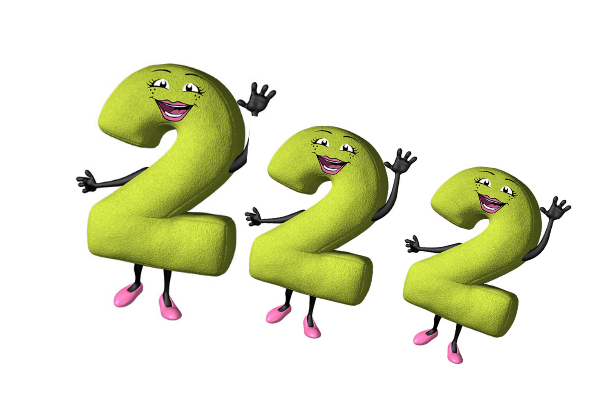## #4. Six Digits Become Three

1- Take a three-digit number, any number you like and write the number twice so that it becomes a six-digit number. For Example, if I take 256 as a three-digit number, I’ll write it as 256256.

2- Now divide the six-digit number by 7

3- Divide the answer you get by 11

4- Divide the answer you get by 13

5- You’ll see the answer you’ll get will always be a three-digit number.

## #5. 11 Rule

You can quickly multiply any two-digit number in your head by 11 and get the result quickly.

1- Think of a two-digit number and sperate it.

3- Now, place the number you get after adding the two digits. If the number you got is greater than 9, carry the one digit to the tens digit.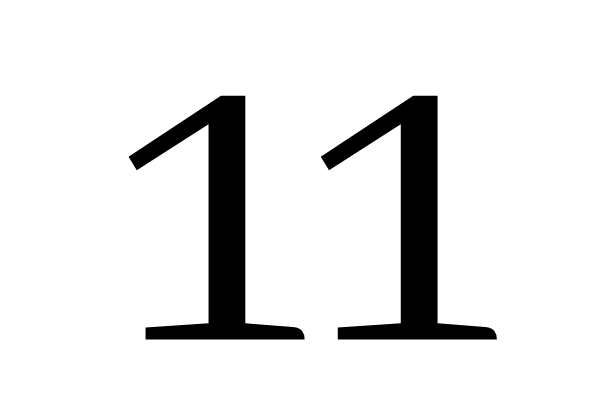So, these were “Maths Tricks That Will Blow Your Mind”. If you know more such tricks do share with us in the comment section. And, also let us know how much fun you find it doing these amazing maths tricks. Don’t forget to mention your views about “Maths Tricks That Will Blow Your Mind”.

Also Try

7 signs that you are a good kisser

5 Zodiac Signs who Always Start the Fight

3 Best Tips to a Healthy Online Relationship

Likes(0)Dislikes(0)